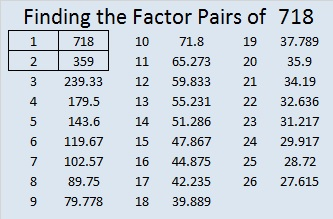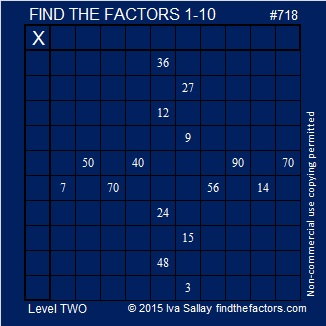# 718 and a Christmas Star

• 718 is a composite number.
• Prime factorization: 718 = 2 x 359
• The exponents in the prime factorization are 1 and 1. Adding one to each and multiplying we get (1 + 1)(1 + 1) = 2 x 2 = 4. Therefore 718 has exactly 4 factors.
• Factors of 718: 1, 2, 359, 718
• Factor pairs: 718 = 1 x 718 or 2 x 359
• 718 has no square factors that allow its square root to be simplified. √718 ≈ 26.795522.Today’s puzzle looks like a Christmas Star.Print the puzzles or type the solution on this excel file: 10 Factors 2015-12-21

——————————————————————————————

Here’s a few facts about the number 718:

178 + 179 + 180 + 181 = 718 making it the sum of 4 consecutive numbers.

718 is the sum of three squares three different ways:

• 3² + 15² + 22² = 718
• 9² + 14² + 21² = 718
• 13² + 15² + 18² = 718

——————————————————————————————This site uses Akismet to reduce spam. Learn how your comment data is processed.